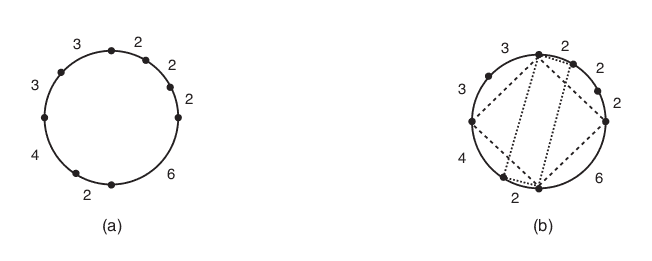### B - Building a Field

##### Languages: MS C# .NET 4.7.2053,GNU G++11 5.1.0 ...

John is a meticulous person. In his farm he built a circular field with some trees planted right at the circumference of the field. Figure (a) below shows the field with the trees.

Now John wants to use a long rope and four of the field trees to demarcate a rectangle using the trees as vertices and the rope as edges. Figure (b) below shows two rectangles that can be demarcated using the trees of the field in figure (a).Given the description of the positions of the trees in John’s circular field, you must determine whether it is possible to demarcate a rectangle using four of the trees as vertices and the rope as edges.

#### Input

The first line contains an integer $N$ ($4 \le N \le 10^5$) indicating the number of trees in the field. Trees are represented as points on a circumference. The second line contains $N$ integers $L_1, L_2, ..., L_N$ ($1 \leq L_i \leq 10^6$ for $i = 1, 2, ..., N$) indicating the arc lengths between each pair of consecutive trees. The arcs are given in counter-clockwise order. The total length of the circumference does not exceed $10^9$.

#### Output

Output a single line with the uppercase letter “Y” if it is possible to demarcate a rectangle using the given trees, and the uppercase letter “N” otherwise.

#### Sample test(s)

Input
8 3 3 4 2 6 2 2 2
Output
Y
Input
4 14 16 15 15
Output
N
Input
6 3 7 7 3 10 10
Output
Y